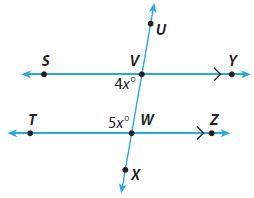Homework Explained - Math Practice 101Dear guest, you are not a registered member. As a guest, you only have read-only access to our books, tests and other practice materials.

As a registered member you can:

Registration is free and doesn't require any type of payment information. Click here to Register.
Go to page:
Chapter 11:Angle Relationships in Parallel Lines and Triangles; Lesson 1:Parallel Lines Cut by a Transversal

### Guided Practice

Use the figure for Exercises 1–4.• Question 1

$$\angle UVY$$ and ____ are a pair of corresponding angles.

•  $$\angle$$
• Question 2

$$\angle WVY$$ and $$\angle VWT$$ are _________ angles.

• Question 3

Find m$$\angle SVW$$.

•  $$^°$$
• Question 4

Find m$$\angle VWT$$.

•  $$^°$$
• Question 5

Vocabulary When two parallel lines are cut by a transversal, _______________ angles are supplementary.

### ESSENTIAL QUESTION CHECK-IN

• Question 6

What can you conclude about the interior angles formed when two parallel lines are cut by a transversal?

• Type below:

Yes, email page to my online tutor. (if you didn't add a tutor yet, you can add one here)